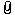Coordinate Frame conversion of coordinatesFrom: Michael Gibson 12 Mar 2012  (4 of 6)5000.4 In reply to 5000.3 Hi Brian, > So the x-axis and y-axis direction vectors used to create the > new frame would be (or could be) in world coordinate frame. Yup, normally those direction vectors are in world coordinates. The origin is also in world coordinates - the whole package of data gives the position and orientation of the frame's coordinate system. > A new y-axis, parallel to the world coordinate y-axis would > have the same vector direction, (0,1,0). Yup that's correct. > The new x-axis vector direction can be calculated from > the two points, mathematically, or with vectorMath... Yup, but the x-axis vector should be one that is at a 90 degree angle to the y axis vector. But it can be pivoted around the x-axis, like for example here in red are different possible x-axis lines that would work with the given green y axis:- Michael Attachments:brian_axis_pivot.jpg